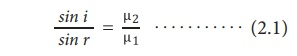Home | | Science 10th Std | Refraction of Light

# Refraction of Light

When a ray of light travels from one transparent medium into another obliquely, the path of the light undergoes deviation. This deviation of ray of light is called refraction.

REFRACTION OF LIGHT

When a ray of light travels from one transparent medium into another obliquely, the path of the light undergoes deviation. This deviation of ray of light is called refraction. Refraction takes place due to the difference in the velocity of light in different media. The velocity of light is more in a rarer medium and less in a denser medium. Refraction of light obeys two laws of refraction.

## 1. First law of refraction:

The incident ray, the refracted ray of light and the normal to the refracting surface all lie in the same plane.

## 2. Second law of refraction:

The ratio of the sine of the angle of incidence and sine of the angle of refraction is equal to the ratio of refractive indices of the two media. This law is also known as SnellŌĆÖs law.┬Ę                 Refractive index gives us an idea of how fast or how slow light travels in a medium. The ratio of speed of light in vacuum to the speed of light in a medium is defined as refractive index ŌĆś┬ĄŌĆÖ of that medium.

┬Ę                 The speed of light in a medium is low if the refractive index of the medium is high and vice versa.

┬Ę                 When light travels from a denser medium into a rarer medium, the refracted ray is bent away from the normal drawn to the interface.

┬Ę                 When light travels from a rarer medium into a denser medium, the refracted ray is bent towards the normal drawn to the interface.

Study Material, Lecturing Notes, Assignment, Reference, Wiki description explanation, brief detail
10th Science : Chapter 2 : Optics : Refraction of Light |